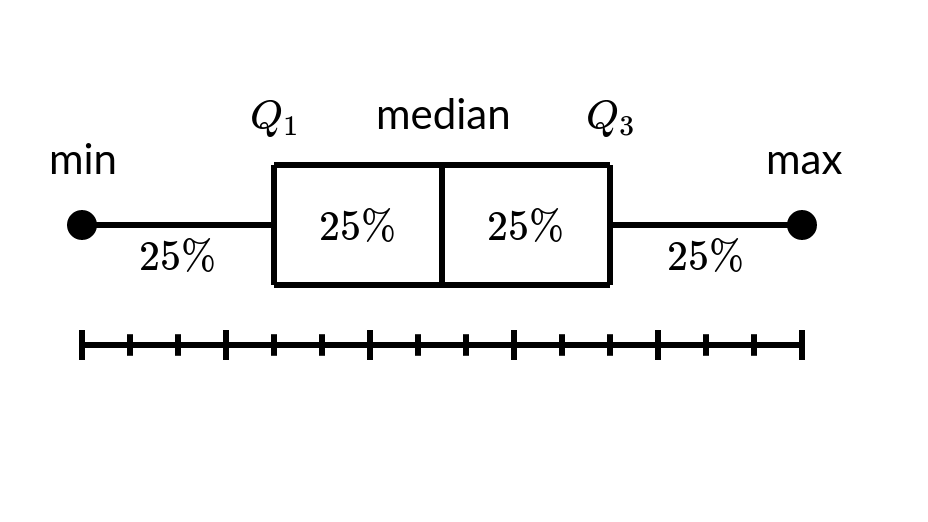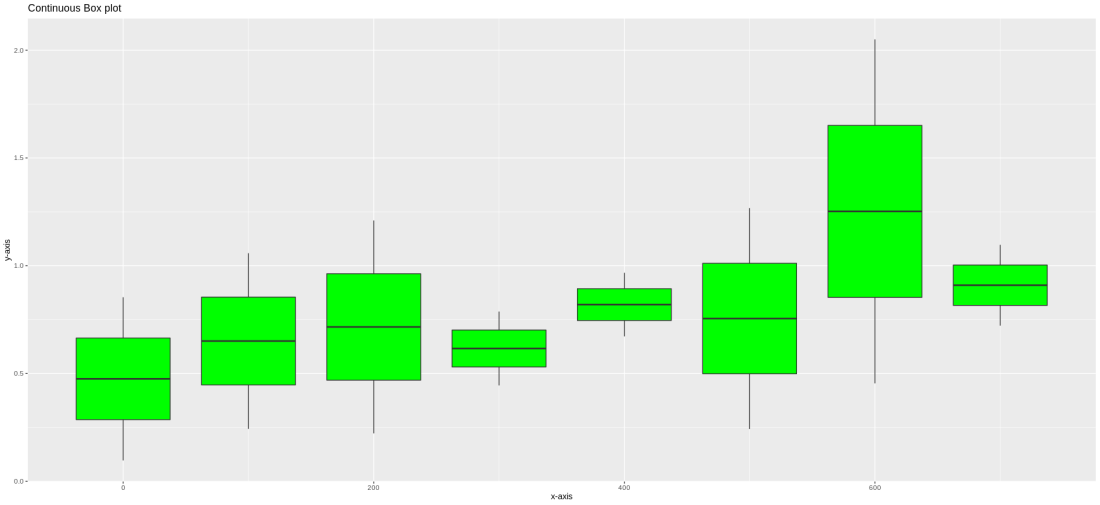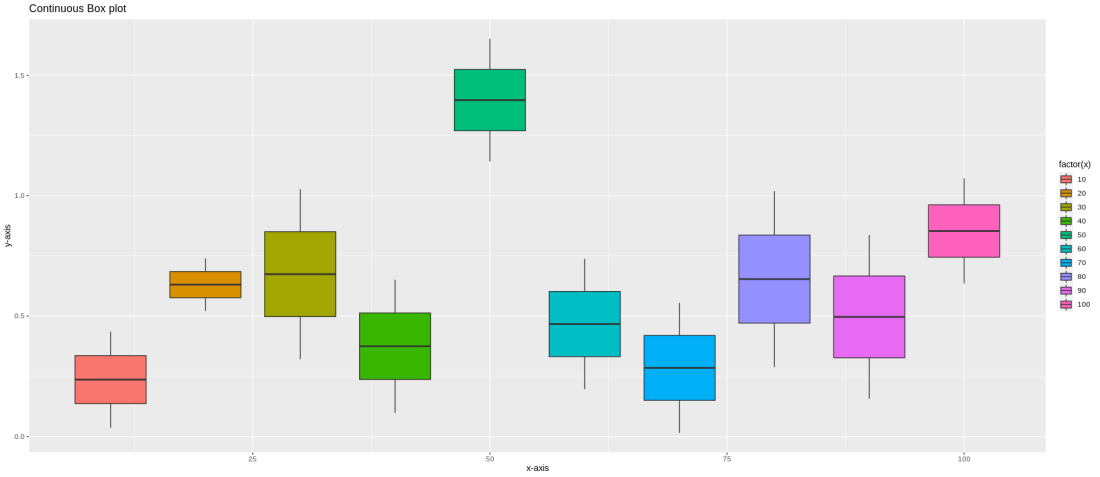# Create boxplot for continuous variables using ggplot2 in R

• Last Updated : 29 Jul, 2021

Box plots are a good way to summarize the shape of a distribution, showing its median, its mean, skewness, possible outliers, its spread, etc. Box-whisker plots are the other name of Box plots.  These plots are mostly used for data exploration. The box plot is the five-number summary, which is the minimum, first quartile, median, third quartile, and maximum.A Box Plot.

The box plot summarizes the distribution of a continuous variable, we draw a box from the first quartile to the third quartile and A vertical line goes through the box at the median, which is the second quartile, splitting the data into two equal percent of 50 below and 50 above. The first quartile(Q1) includes the first 25 percent of the data, and the third quartile(Q3) includes 75 percent of the data.

Using the geom_boxplot() function from ggplot2 package from R, we can create a simple box plot and also a box plot from the continuous variable :

Syntax: geom_boxplot(mapping = NULL, data = NULL,position = “dodge”, outlier.colour = NULL, outlier.shape = 19, outlier.size = 1.5, outlier.stroke = 0.5, …)

Parameters:

• mapping: In this mapping we provide the column name as an argument to map onto the plot. The default mapping in geom_boxplot is NULL.
• data: This parameter sets the data frame to be used.
• position: position argument specify how the boxplot will be placed during the visual representation of the figure. The default value of the position is dodge.
• outlier.colour: Used to specifies  specifies the default colour of the outlier.
• outlier.shape: Used to specifies  specifies the default colour of the outlier.
• outlier.size: Used to specifies the default size of the outlier.
• outlier.stroke:  we can hide the outliers from chart using the outlier.shape = NA it only hides the outlier, it doesn’t remove the outlier.

To create a box plot for a continuous variable, first, install the necessary packages for plotting box plots and then create or load the dataset for which we want to plot the box plot. Plot the box plot using geom_boxplot() function like a regular boxplot.

Example 1:

## R

 `# loading library``library``(ggplot2)``  ` `# creating random dataset``data <- ``data.frame``(y=``abs``(``rnorm``(16)),``                   ``x=``rep``(``c``(0,100,200,300,400,``                           ``500,600,700),``                         ``each=2))``  ` `# creating the box plot``ggplot``(data, ``aes``(x, y, group=x)) +``    ` `# plotting the box plot with green color``geom_boxplot``(fill=``"green"``) +``    ` `# adding x-axis label``xlab``(``"x-axis"``) +``    ` `# adding y-axis label``ylab``(``"y-axis"``) +``    ` `# adding title``ggtitle``(``"Continuous Box plot "``)`

Output:Box plot

Example 2:

## R

 `# creating box plot for continuous variable   ``# loading library``library``(ggplot2)` `# creating random dataset``data <- ``data.frame``(y=``abs``(``rnorm``(20)),``                   ``x=``rep``(``c``(10,20,30,40,50,60,``                           ``70,80,90,100),``                         ``each=2))` `# creating the box plot``ggplot``(data, ``aes``(x, y, fill=``factor``(x))) +`` ` `  ``# plotting the box plot with green color``  ``geom_boxplot``() +``  ` `  ``# adding x-axis label``  ``xlab``(``"x-axis"``) +``  ` `  ``# adding y-axis label``  ``ylab``(``"y-axis"``) +``  ` `  ``# adding title``  ``ggtitle``(``"Continuous Box plot "``)`

Output:Colored Box plot

My Personal Notes arrow_drop_up